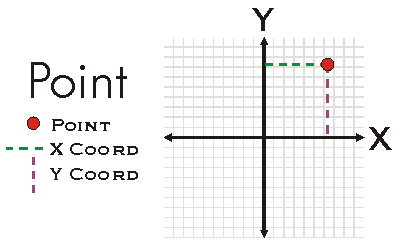ArcObjects Library Reference (Geometry)

# IPoint.Y Property

The Y coordinate.

```[Visual Basic .NET]
Public Property Y As Double```
```[C#]
public double Y {get; set;}```
```[C++]
HRESULT get_Y(
double* Y
);```
```[C++]
HRESULT put_Y(
double Y
);```
```[C++]
Parameters
Y [out, retval]
Y is a parameter of type double
Y
Y is a parameter of type double
```

#### Product Availability

Available with ArcGIS Engine, ArcGIS Desktop, and ArcGIS Server.

#### Description

Returns and Sets the Y coordinate of the Point.  The Y coordinate is the vertical position of the point.

#### Remarks[C#]

// The following example shows how to set the x,y,z,m properties
// for a point. Note that you need to tell the point to be
// M- and Z-aware.
public void DisplayCoordinates()
{
IPoint point = new PointClass();
point.PutCoords(100, 100);
IMAware mAware = point as IMAware;
mAware.MAware = true;
IZAware zAware = point as IZAware;
zAware.ZAware = true;
point.Z = 50;
point.M = 10;
System.Windows.Forms.MessageBox.Show(point.X + "," + point.Y + "," + point.Z + "," + point.M);
}